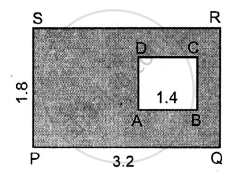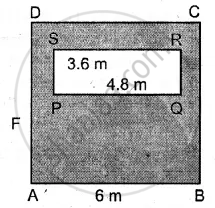# In Each of the Following Cases, Abcd is a Square and Pqrs is a Rectangle. Find, in Each Case, the Area of the Shaded Portion. (All Measurements Are in Metre). - Mathematics

Sum

In each of the following cases, ABCD is a square and PQRS is a rectangle. Find, in each case, the area of the shaded portion.
(All measurements are in metre).

(i)(ii)#### Solution

(i)

= Area of the rectangle PQRS – Area of square ABCD
= 3.2 x 1.8 – (1.4)2 (∵ PQ = 3.2 and PS = 1.8) Side of square AB = 1.4
= 5.76 – 1.96 = 3.80 = 3.8 m2

(ii)

Area of the shaded portion = Area of square ABCD – Area of rectangle PQRS
= 6 x 6 – (3.6) (4.8) = 36 – 17.28 = 18.72 m2

Is there an error in this question or solution?
Chapter 20: Area of a Trapezium and a Polygon - Exercise 20 (B) [Page 227]

#### APPEARS IN

Selina Concise Mathematics Class 8 ICSE
Chapter 20 Area of a Trapezium and a Polygon
Exercise 20 (B) | Q 13 | Page 227
Share